# Two-Compartment Open Model: Intravenous Bolus Administration

| Home | | Biopharmaceutics and Pharmacokinetics |

## Chapter: Biopharmaceutics and Pharmacokinetics : Compartment Modelling

The model can be depicted as shown below with elimination from the central compartment.

Two-Compartment Open Model

The model can be depicted as shown below with elimination from the central compartment.After the i.v. bolus of a drug that follows two-compartment kinetics, the decline in plasma concentration is biexponential indicating the presence of two disposition processes viz. distribution and elimination. These two processes are not evident to the eyes in a regular arithmetic plot but when a semilog plot of C versus t is made, they can be identified (Fig. 9.12). Initially, the concentration of drug in the central compartment declines rapidly; this is due to the distribution of drug from the central compartment to the peripheral compartment. The phase during which this occurs is therefore called as the distributive phase. After sometime, a pseudo-distribution equilibrium is achieved between the two compartments following which the subsequent loss of drug from the central compartment is slow and mainly due to elimination. This second, slower rate process is called as the post-distributive or elimination phase. In contrast to the central compartment, the drug concentration in the peripheral compartment first increases and reaches a maximum. This corresponds with the distribution phase. Following peak, the drug concentration declines which corresponds to the post-distributive phase (Fig.9.12).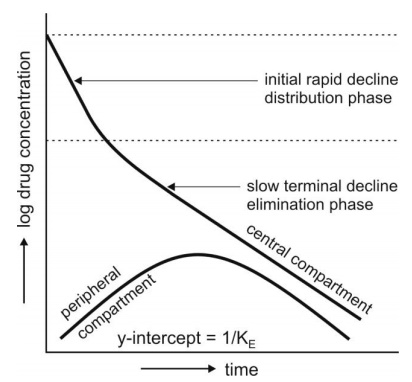Fig. 9.12. Changes in drug concentration in the central (plasma) and the peripheral compartment after i.v. bolus of a drug that fits two-compartment model.

Let K12 and K21 be the first-order distribution rate constants depicting drug transfer between the central and the peripheral compartments and let subscript c and p define central and peripheral compartment respectively. The rate of change in drug concentration in the central compartment is given by: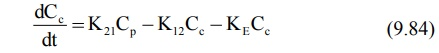Extending the relationship X = VdC to the above equation, we have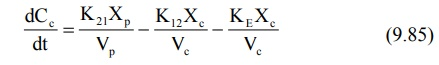where Xc and Xp are the amounts of drug in the central and peripheral compartments respectively and Vc and Vp are the apparent volumes of the central and the peripheral compartment respectively. The rate of change in drug concentration in the peripheral compartment is given by: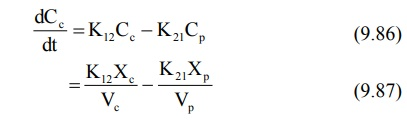Integration of equations 9.85 and 9.87 yields equations that describe the concentration of drug in the central and peripheral compartments at any given time t: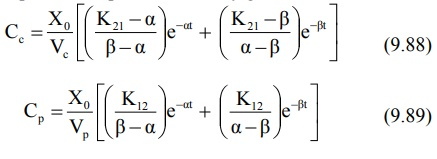where Xo = i.v. bolus dose, α and β are hybrid first-order constants for the rapid distribution phase and the slow elimination phase respectively which depend entirely upon the first-order constants K12, K21 and KE.

The constants K12 and K21 that depict reversible transfer of drug between compartments are called as microconstants or transfer constants. The mathematical relationships between hybrid and microconstants are given as:

α + β = K12 + K21 + KE                     (9.90)

αβ = K21KE                    (9.91)

Equation 9.88 can be written in simplified form as:

Cc = Ae –αα + Be βt                (9.92)

Cc = Distribution exponent Elimination exponent

where A and B are also hybrid constants for the two exponents and can be resolved graphically by the method of residuals.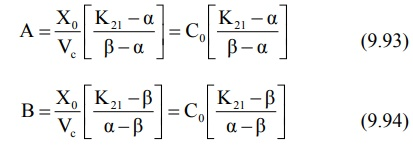where Co = plasma drug concentration immediately after i.v. injection.

Method of Residuals: The biexponential disposition curve obtained after i.v. bolus of a drug that fits two compartment model can be resolved into its individual exponents by the method of residuals. Rewriting the equation 9.92:

Cc = Ae -αα + Be -βt                     (9.92)

As apparent from the biexponential curve given in Fig. 9.12., the initial decline due to distribution is more rapid than the terminal decline due to elimination i.e. the rate constant α >> ß and hence the term eαt approaches zero much faster than does eβt. Thus, equation 9.92 reduces to: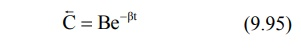In log form, the equationwhere C = back extrapolated plasma concentration values. A semilog plot of C versus t yields the terminal linear phase of the curve having slope –β/2.303 and when back extrapolated to time zero, yields y-intercept log B (Fig. 9.13.).The t½ for the elimination phase can be obtained from equation t½ = 0.693/β.

Subtraction of extrapolated plasma concentration values of the elimination phase (equation 9.95) from the corresponding true plasma concentration values (equation 9.92) yields a series of residual concentration values Cr.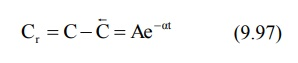In log form, the equation becomes:A semilog plot of Cr versus t yields a straight line with slope –α/2.303 and Y-intercept log A (Fig. 9.13).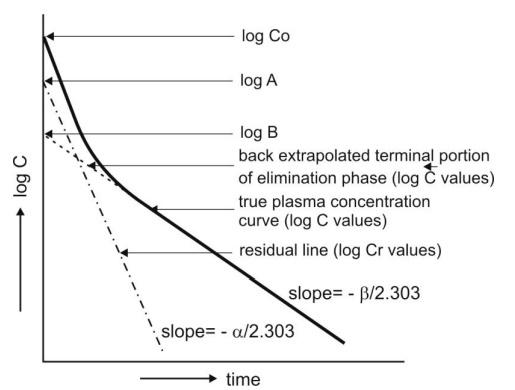Fig. 9.13. Resolution of biexponential plasma concentration-time curve by the method of residuals for a drug that follows two-compartment kinetics on i.v. bolus administration.

Assessment of Pharmacokinetic Parameters: All the parameters of equation 9.92 can be resolved by the method of residuals as described above. Other parameters of the model viz. K12, K21, KE, etc. can now be derived by proper substitution of these values.

C0 = A + B                       (9.99)It must be noted that for two-compartment model, KE is the rate constant for elimination of drug from the central compartment and β is the rate constant for elimination from the entire body. Overall elimination t½ should therefore be calculated from β.

Area under the plasma concentration-time curve can be obtained by the following equation: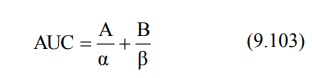The apparent volume of central compartment Vc is given as: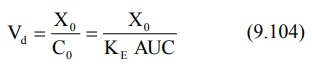Apparent volume of peripheral compartment can be obtained from equation: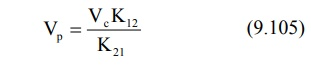The apparent volume of distribution at steady-state or equilibrium can now be defined as: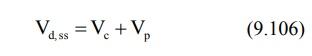It is also given as: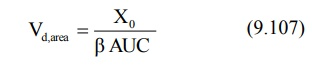Total systemic clearance is given as: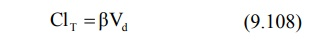The pharmacokinetic parameters can also be calculated by using urinary excretion data: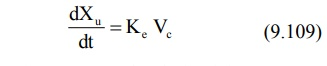An equation identical to equation 9.92 can be derived for rate of excretion of unchanged drug in urine: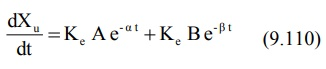The above equation can be resolved into individual exponents by the method of residuals as described for plasma concentration-time data.

Renal clearance is given as:

ClR Ke Vc                     (9.111)